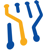Unplugged Viz High-performance scientific visualization isn't just for the workstation anymore
Durwella.Unplugged.Viz.GridGeometry Class Reference

Helper methods for generating grid geometries. More...

## Static Public Member Functions

static int[] GenerateTriangleStripIndices (int vertexRows, int vertexColumns, bool wrap=false)
Generate triangle strip indices for a grid with given numbers of rows and columns. Assumes the vertices are laid out in rows, then columns; for example: More...

static float[,,] GenerateTextureCoordinates (int rows, int columns, float uMax=1, float vMax=1)
Generate texture coordinates for a grid with given numbers of rows and columns. More...

## Detailed Description

Helper methods for generating grid geometries.

## Member Function Documentation

 static float [,,] Durwella.Unplugged.Viz.GridGeometry.GenerateTextureCoordinates ( int rows, int columns, float uMax = `1`, float vMax = `1` )
static

Generate texture coordinates for a grid with given numbers of rows and columns.

Returns
The texture coordinates: a float[rows, columns, 2] with values between 0 and 1 mapping texture indices to vertices.
Parameters
 rows The vertex row count. columns The vertex column count. uMax The proportion of the texture rows for which their are data. vMax The proportion of the texture columns for which their are data.
 static int [] Durwella.Unplugged.Viz.GridGeometry.GenerateTriangleStripIndices ( int vertexRows, int vertexColumns, bool wrap = `false` )
static

Generate triangle strip indices for a grid with given numbers of rows and columns. Assumes the vertices are laid out in rows, then columns; for example:

0 1 2 3

4 5 6 7

8 9 0 1

2 3 4 6

Returns
The triangle strip indices.
Parameters
 vertexRows The vertex row count. vertexColumns The vertex column count. wrap If set to `true` wrap the indices around the model.

The documentation for this class was generated from the following file:
• GridGeometry.cs